Tuning the Elo ratings: The K-factor and home field advantage

The Elo rating system is quite simple, and therefore easy implement. In football, FIFA uses is in its womens rankings and the well respected website fivethirtyeight.com also uses Elo ratings to make predictions for NBA and NFL games. Another cool Elo rating site is clubelo.com.

Three year ago I posted some R code for calculating Elo ratings. Its simplicity also makes it easy to modify and extend to include more realistic aspects of the games and competitions that you want to make ratings for, for example home field advantage. I suggest reading the detailed description of the clubelo ratings to get a feel of how the system can be modified to get improved ratings. I have also discussed some ways to extend the Elo ratings here on this blog as well.

If you implement your own variant of the Elo ratings it is necessary to tune the underlying parameters to make the ratings as accurate as possible. For example, a too small K-factor will give ratings that update too slow. The ratings will not adapt well to more recent developments. Vice versa, a too large K-factor will put too much weight on the most recent results. The same goes for the extra points added to the home team rating to account for the home field advantage. If this is poorly tuned, you will get poor predictions.

In order to tune the rating system, we need a way to measure how accurate the ratings are. Luckily the formulation of the Elo system itself can be used for this. The Elo system updates the ratings by looking at the difference between the actual results and the results predicted by the rating difference between the two opposing teams. This difference can be used to tune the parameters of the system. The smaller this difference is, the more accurate are the predictions, so we want to tune the parameters so that this difference is as small as possible.

To formulate this more formally, we use the following criterion to assess the model accuracy:

$$\sum_i[ (exp_{hi} – obs_{hi})^2 + (exp_{ai} – obs_{ai})^2 ]$$

where $$exp_{hi}$$ and $$exp_{ai}$$ are the expected results of match i for the home team and the away team, respectively. These expectations are a number between 0 and 1, and is calculated based on the ratings of the two teams. $$obs_{hi}$$ and $$obs_{ai}$$ are the actual result of match i, encoded as 0 for loss, 0.5 for draw and 1 for a win. This criterion is called the squared error, but we will use the mean squared error.

With this criterion in hand, we can try to find the best K-factor. Using data from the English premier league as an example I applied the ratings on the match results from the January 1st 2010 to the end of the 2014-15 season, a total of 2048 matches. I tried it with different values of the K-factor between 7 and 25, in 0.1 increments. Then plotting the average squared error against the K-factor we see that 18.5 is the best K-factor.The K-factor I have found here is, however, probably a bit too large. In this experiment I initialized the ratings for all teams to 1500. This includes the teams that was promoted from the Championship. A more realistic rating system would initialize these teams with a lower rating, perhaps be given the ratings from the relegated teams.

We can of course us this strategy to also find the best adjustment for the home field advantage. The simple way to add the home field advantage is to add some additional points to the ratings for the home team. Here I have used the same number of points in all matches across all season, but different strategies are possible. To find the optimal home field advantage I applied the Elo ratings with K=18.5, using different home field advantages.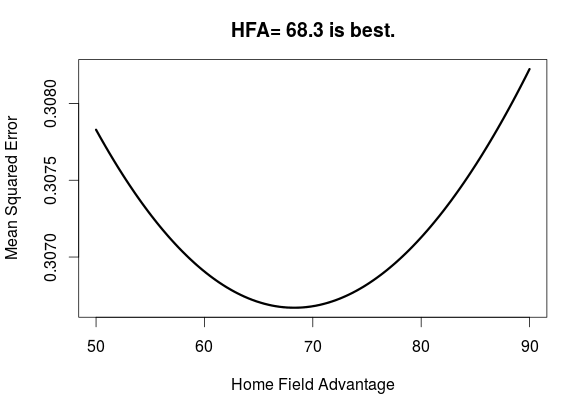From this plot we see that an additional 68.3 points is the optimal amount to add to the rating for the home team.

One might wonder if finding the best K-factor and home field advantage independent of each other is the best way to do it. When I tried to find the best K-factor with the home field advantage set to 68, I found that the best K was 19.5. This is a bit higher than when the home field advantage was 0. I tried to find the optimal pair of K and home field advantage by looking over a grid of possible values. Plotting the accuracy of the ratings against both K and the home field advantage in a contour we get the following: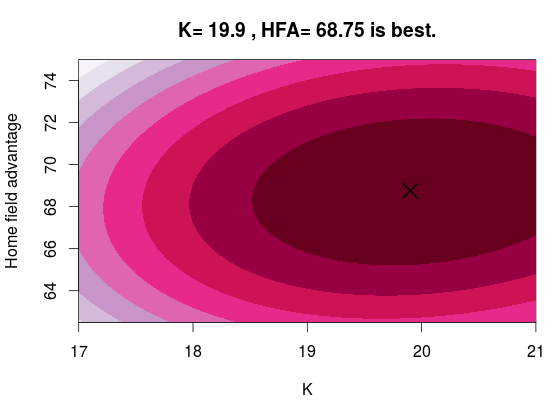The best K and home field advantage pair can be read from the plot, both of which is a bit higher than the first values I found.

Doing the grid search can take a bit of time, especially if you don’t narrow down the search space by doing some initial tests beforehand. I haven’t really tried it out, but alternating between finding the best K-factor and home field advantage and using the optimal value from the previous round is probably going to be a reasonable strategy here.

My predictions for the 2016-17 Premier League

This year I am participating in Simon Gleave‘s Premier League prediction competition. It is an interesting initiative, as both statistical models and and more informal approaches are compared.

Last time I participated in something like this was midway trough the last Premier League season for statsbomb.com’s compilation. This time, however, the predictions are made before the first match has been played. To be honest, I think it is futile to try to model and predict an unplayed season since any model based only on previous results will necessarily reproduce what has already happened. This approach will work OK for predicting the result of the next couple of matches midway trough a season, but making predictions for the start of a season is really hard since the teams have brought inn some new players and gotten rid of other and perhaps also changed managers and so on. And not to forget that we also try predict results 9 months into the future.

When May comes and my predictions are completely wrong, I am not going to be embarrassed.

Last time I wanted to use the Conway-Maxwell-Poisson model, but I did not get it to work when I included data from several seasons plus data from the Championship. I still did not get it to work properly, but this time I tried a different approach to estimate the parameters. I ended up using a two-step approach, where I first estimate the attack and defense parameters with the independent Poisson model, and then, keeping those parameters fixed, I estimated the dispersion parameter by itself.

To fit the model I used Premier League data from the 2010-11 season to the 2015-16 season. I also included data from the 2015-16 season of the Championship (including the playoff) to be able to get some information on the promoted teams. I used the Dixon-Coles weighting scheme with $$\xi = 0.0019$$. I used a separate parameter for home field advantage for Premier League and the Championship. I also used separate dispersion parameters for the two divisions.

I estimated the dispersion parameter for the Premier League to be 1.103, about the same as I previously estimated in some individual Premier League seasons, indicating some underdispersion in the goals. Interestingly, the dispersion parameter for the Championship was only 1.015.

Anyway, here are my projected league table with expected (or average) point totals. This is completely based on the model, I have not done any adjustments to it.

Team Points
Manchester City 73.70
Arsenal 69.73
Leicester City 64.12
Manchester United 63.95
Chelsea 63.84
Tottenham 62.53
Southampton 60.51
Liverpool 60.37
Everton 51.48
West Ham 51.12
Middlesbrough 46.30
Swansea 44.59
Burnley 44.20
Stoke City 42.99
Hull 42.49
Crystal Palace 41.33
Watford 41.23
Sunderland 39.83
West Bromwich Albion 39.21
Bournemouth 36.37

The Norwegian election survey: Voter turnout across generations and age groups

In the last post I used data from the Norwegian election survey to look at how party preferences changed between generations. One thing I didn’t look at was if there was any differences in participation between the generations. While the Norwegian elections generally has a high turnout, the general trend has been a decline. Some numbers on voter turnout are available from Statistics Norway, and a plot of turnout for the national elections for parliament and the local elections show that this is especially true for the local elections. For the parliament elections there seems to be a sudden drop in turnout at the 1993 election. Before that the general turnout was somewhere between 80% and 85%. From 1993 and onwards it has been somewhere between 75% and 80%.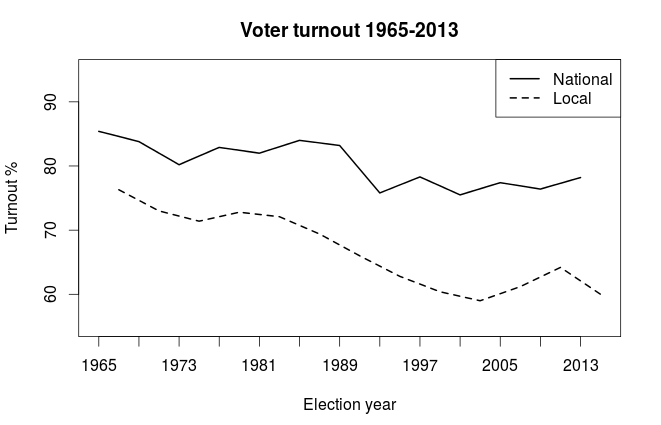This time I decided to only look at the surveys done in connection with the parliamentary elections. This was to avoid much clutter with the differences between the local and national elections. After I gathered the data from the elections surveys using the web analysis tool at the web page for Norwegian Center For Research Data (see the link above), I plotted the voter turnout for each election for each birth cohort (in 7-year groups). In the plot is each election represented by a line. The same thing I did in the previous post, in other words.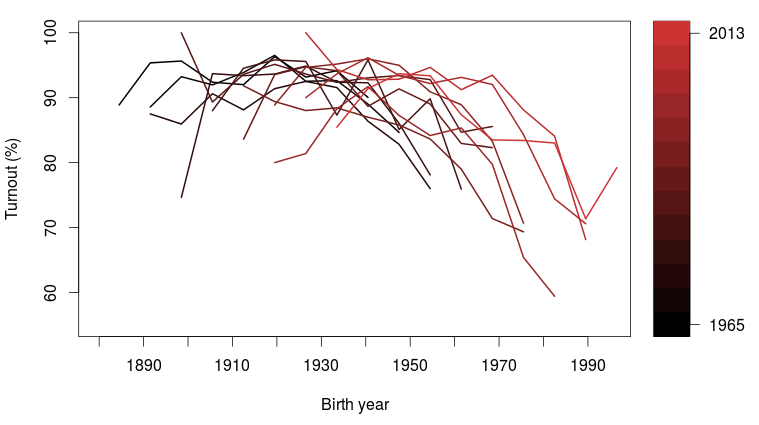We clearly see a trend in which younger generations, those born after about 1955, are less likely to vote. But there is also a clear indication that the young voters, when they get older, are more likely to vote. This trend can for example be seen for the 1970-generation. The earliest generations where this group could vote are those lines where the line ends in about 1970. In the earliest elections where this group could vote, more than 25% did not vote, but in the more recent elections only 10% of this generation did not vote.

Also notice how in each election, the oldest group also seem to have a tendency to not vote. This could perhaps be explained by the older population generally has poorer health and will therefore not prioritize to get out to vote. But I also suspect this is partially explained by random variation, as the oldest birth groups have relatively few respondents in the surveys.

We can plot the turnout by age instead of birth year to get a better view of the differences between age groups. Here I used 5-year groups instead of 7. In this plot the lines do seem to align a bit better.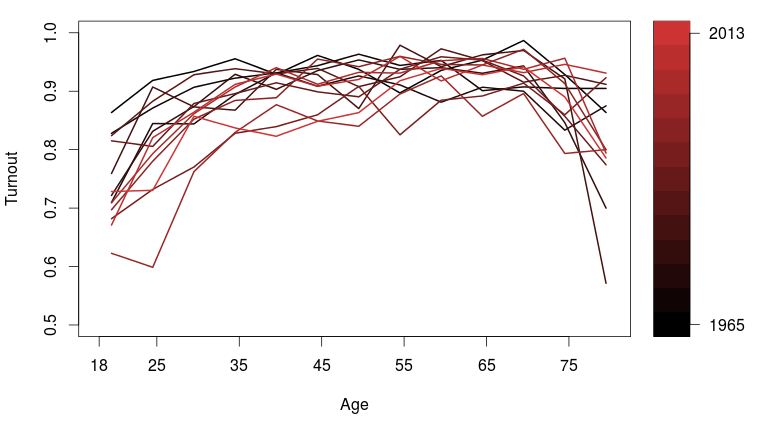Still another figure we could do is to plot the turnout for different age groups, and then see how this has changed from election to election. Here I have plotted only two age groups, those 25 or younger, and those older than 25. Also shown is the national turnout, which is not from the election survey, but are the official turnout numbers. This is the same as in the first plot above.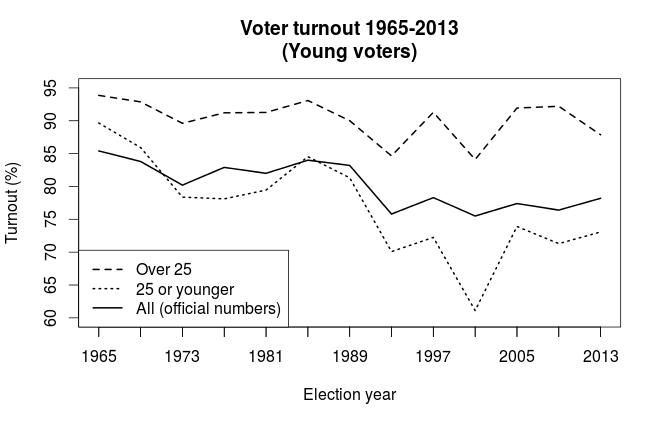We see again that the young voters have lower turnout than the older ones, which by now should be no surprise. In addition, the difference between the young and the old seem follow each other between the elections to a large degree, going up and down in a similar pattern, but it also become noticeably wider from the 1993 election. From just looking at this plot, it could seem as if the lower turnout among the young could explain a lot of the decrease that happened in the 1993 election, but keep in mind that the younger group is a relatively small group. Not pictured in the plot is the uncertainty of the estimates, which gives the unreasonable results in the 1965 and 1985 elections, where both the young and old have higher turnout (as measured by the survey) than the official numbers.

So from looking at these plots, it seems like when people where born, what age you are and which election it is influence whether you vote or not. But the effect of these three aspects is hard, if not impossible, to untangle. The reason for this is simple: How old you are is fully determined by when you are and when you were born. You can of course turn it around and say the same for the two other aspects: If you know two of them, you also know the third. From a modeling point of view this dependency makes it hard to put these three variables in a regression model, but there are some literature out there on how this kind of Age-Period-Cohort analysis (as it is called) could be done.

But does this mean we can’t really learn anything from it? I think we can. The kind of analysis like the one I have done here is of course rather informal and descriptive, no p-values or effect sizes or stuff like that, but I think it is clear that age plays an important role. The third plot, with age on the horizontal axis, looks much nicer than the second plot, with birth year on the horizontal. The lines align rather nicely. We can also see this in the cohort plot, where the 1970-generation had a low turnout in the first elections they could participate in, but in the more recent elections they participate as much as those born before that.

Whether the changes in participation among the young over time is a period effect or a cohort effect is more difficult to say. It seems to covary with the general trend, but it also has it’s own component. This does not seem to play a large role, except perhaps a change at the 1985 election (or among those born in the 1960’s, depending on your view).

Calculate the ranked probability score in R

I was asked in the comments for the R code for the ranked probability score, so instead of posting it deep down in the comments I thought I’d post it as a proper blog instead. The ranked probability score (RPS) is a measure of how similar two probability distributions are and is used as a way to evaluate the quality of a probabilistic prediction. It is an example of a proper scoring rule.

The RPS was brought to my attention in the paper Solving the problem of inadequate scoring rules for assessing probabilistic football forecasting models by Constantinou and Fenton. In that paper they argue that the RPS is the best measure of the quality of football predictions when the predictions are of the type where you have probabilities for the outcome (home win, draw or away win). The thing about the RPS is that it also reflects that an away win is in a sense closer to a draw than a home win. That means that a higher probability predicted for a draw is considered better than a higher probability for home win if the actual result is an away win.

You can also find some more details at the pena.lt/y blog.

The following R function takes two arguments. The first argument (predictions) is a matrix with the predictions. It should be laid out so that each row is one prediction, laid out in the proper order, where each element is a probability and each row sum to 1. The second argument (observed) is a numeric vector that indicates which outcome that was actually observed.

For assessing football predictions the predictions matrix would have three columns, with the probabilities for the match ordered as home, draw and away (or in the opposite order).

rankProbScore <- function(predictions, observed){
ncat <- ncol(predictions)
npred <- nrow(predictions)

rps <- numeric(npred)

for (rr in 1:npred){
obsvec <- rep(0, ncat)
obsvec[observed[rr]] <- 1
cumulative <- 0
for (i in 1:ncat){
cumulative <- cumulative + (sum(predictions[rr,1:i]) - sum(obsvec[1:i]))^2
}
rps[rr] <- (1/(ncat-1))*cumulative
}
return(rps)
}

The Norwegian election survey: Voting patterns across generations.

Predicting election outcomes has in the recent years been a popular activity among data analytics. I guess you all know how Nate Silver became known for his predictions in the United States elections. Next year is Norwegian election for parliament and I have been thinking about maybe making an attempt at predicting the results when that time comes. There are already some people in Norway doing this, like the pollofpolls.no website and the Norwegian Computing Center.

In the meantime I decided to take a look at some historical data. After each election in Norway a large survey (1500-2000+ respondents) is carried out in an attempt to figure out why people voted what they did. This has been going on since the 1950’s and includes both local and national elections. The data from the surveys are available online from the Norwegian Center For Research Data. Only raw data from the oldest surveys are available for immediate download, but the online analytics tool at the website can be used to create simple tabulations of the all variables in the raw data and the results can downloaded as spreadsheets.

The obvious thing to look at in data like these are if there are any correlations between voting patterns and demographic variables. Gender, income and geography are obvious ones, but they are pretty boring boring, so I didn’t want to look at those. Instead I decided to look at what the relationship between birth year and party preference were.

I used the online tool and tabulated year of birth (or age, if that was the only available) against which party each respondent voted and downloaded the raw numbers. I did this for each survey all the available national elections, and the local elections in this millennium. This gave data on 17 elections from 1957 to 2013. I then cleaned the data a bit, threw out the category of parties termed “others” (usually less than 2% of the votes), calculated the birth year from age where necessary, and a bunch of other small details. With 1500+ respondents, about 70 birth years in each election and about 7 parties gives about 3 to 4 respondents in each cell, on average. Some parties have much lower support, so these tend to have even lower counts. It was therefore necessary to aggregate the birth years into groups. After some experimenting, I ended up by grouping them in 7 year bins.

What makes birth year more interesting to look at than age is that it gives a window back in time. By looking at age only you get a range of ages from 18 to about 90, but when you look at this data from the birth cohort view you can see 150+ years back in time. The oldest respondent in the data set was born in 1865.

Okay, on to some plots. We can start out with the the support for the Labour Party which has been the most popular party in the time after WWII.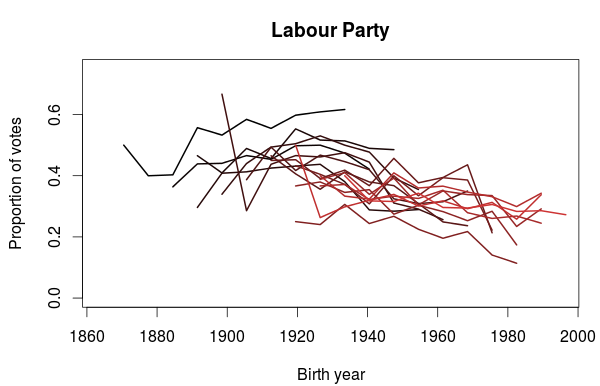Each line in this plot is one election. The colors goes from black (the 1957 election) to red (the 2013 local election). We see that the general trend is that the Labour Party have most support among voters born before 1950, and that there is a decline among younger generations. We also see a trend where they are not as popular as they used to be in the 1960’s and 70’s, which is also seen in the generations born in the pre-1950’s cohorts. The dark red line at the bottom is the 2001 election, where the they did their worst election since the 1920’s.

So let’s take a look at the support for the Conservative Party, the second most popular party.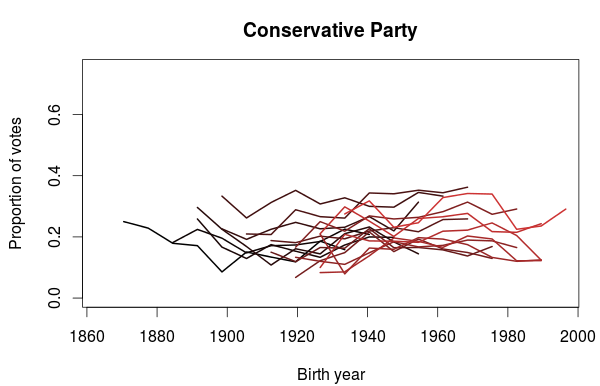Unlike the Labour Party, there does not seem to be any generational trend at all. The Conservatives has usually received between 15-25% of the votes, except at a period in the 1980’s, where they received 30%.

The next party up is the Progress Party, which is currently in a coalition cabinet with the Conservative Party. The first election they participated in was the 1973 election, so the birth year series don’t go as far back as the other parties.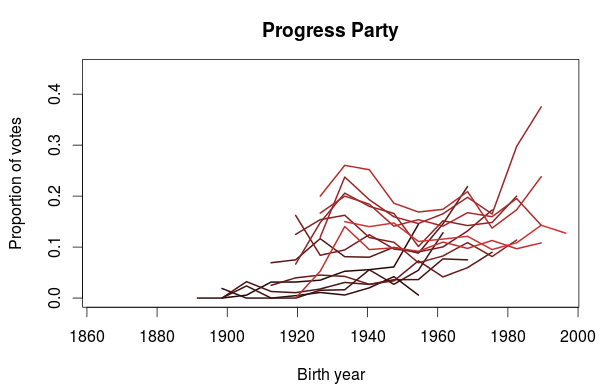I think this plot is very interesting. It looks like the Progress Party is popular among people born in the 1930’s but also among the young voters. Notice how the rightmost part of each lines tend to point upwards. The 1930’s birth trend does not however seem to be present in the earliest elections (those with the darkest lines), but the popularity among the youngest part of the election cohort is there.

The support for the Christian Democratic Party also show some interesting trends. In the plot below we clearly see that they get a sizable portion of their votes from people born before 1940’s. Also noticeable are the two elections in the 1990’s where they did particularly well, where a lot of younger voters also voted for them. Does it also look like a small bump in popularity for voters born in the 1980’s? It could be just a coincidence, so it will be interesting to see if this appears in the next election as well.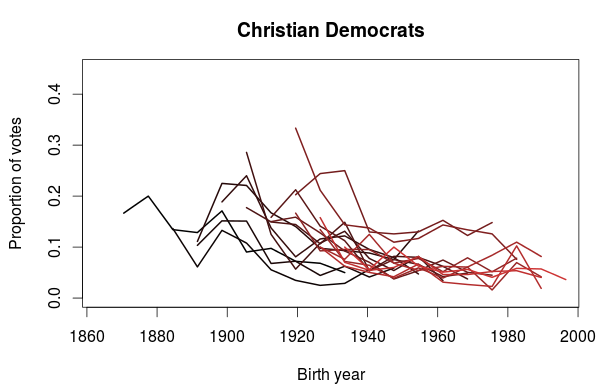The last plot I want to show is for the Socialist Left Party. What this plot clearly shows is that the Socialist Party is more popular among the younger generations than the older. This does not mean we can extrapolate this into future elections and predict an increased popularity. On the contrary, we also see that their decreasing popularity since their peak in 2001 also applies to the younger generations. One could speculate that some of the younger voters have left the Labour Party in favor of the Socialist Party, and that will be the topic in a future blog post.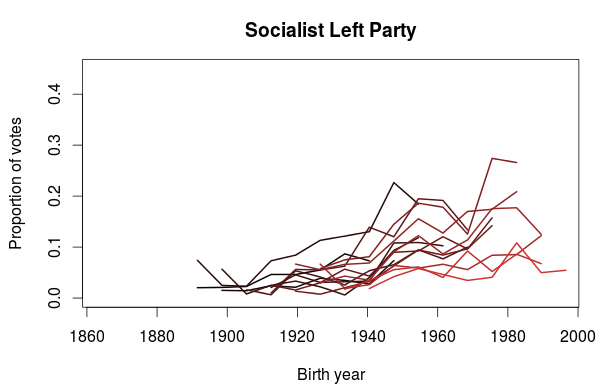My predictions for the rest of the Premier League season

A couple of weeks ago Constantinos Chappas asked on twitter for predictions for the results of the remaining season of English Premier League:

I had been thinking about posting some predictions about the Premier League around new years, since this season is really exciting and it would be a great opportunity to see how well my models would cope with everything that is currently going on. I have never posted any predictions before, so this will surely be an interesting experience. And I thought Chappas’ initiative was really interesting, so that surely gave me a nice reason to come trough.

Today Chappas posted the combined results from all 15 participants so I thought I could share some of the details behind my contribution.

I originally wanted to use the Conway-Maxwell model I have written about recently, but I had some problems with the estimation procedure, so I instead used a classic Poisson model. I used data on Premier League and Championship results going back to the 2011-12 season. By including data from the Champoionship I hope to get better predictions, like I have demonstrated before. Since I used data from a long time back I used the Dixon-Coles weighting scheme, which make more recent games have a greater impact on the predictions. The weighting parameter $$\xi$$ was set to 0.0019, which gives a bit more weight on more recent games than the 0.0018 I found to be most optimal earlier.

I fitted the model and calculated the probabilities for the remaining games of the season. From these probabilities I simulated the rest of the season ten thousand times. From these simulations we can get the probabilities and expectations for the end of season results.

So how do I predict the league table will look like at the end of the season?

Team Points
Manchester City 75.7
Arsenal 75.2
Tottenham 65.6
Leicester City 64.8
Manchester United 64.3
Liverpool 58.2
West Ham 56.1
Chelsea 54.7
Everton 53.7
Crystal Palace 53.7
Stoke City 52.9
Watford 51.9
Southampton 50.6
West Bromwich Albion 45.8
Norwich City 43.7
Bournemouth 42.9
Swansea City 40.9
Newcastle 34.5
Sunderland 31.5
Aston Villa 23.1

Although I predict 0.2 points more for Manchester City than Arsenal, the probabilities for both of them to win is 47.0%. I also give Tottenham a 2.3% chance, Leicester 2.1% and Manchester United a 1.5%. At last, Liverpool have a 0.1% chance. The other teams have a chance less than 0.04%.

I will come back with an update with my entire table with probabilities for all positions for all teams.

The underdispersed Conway-Maxwell Poisson distribution and goal differences

I have unfortunately not had the time to look more closely at the performance for the underdispersed count distributions that I in my last post found to be useful for predicting football results. Here I am taking a quick look into how the Conway-Maxwell distribution (COM) influences the predicted goal differences compared to the Poisson distribution.

Using data from the 2010-11 Premier League season I fitted the both the Poisson model and the COM model. The estimated dispersion parameter for the COM model indicated that there was less variability in the actual goals scored than implied by the Poisson distrubtion. I used the code I posted here to compute the probability distributions for the goal-differences for five matches in the season.

Let’s first look at the goal difference distribution for Arsenal playing at home against Manchester City. Both teams were in the top of the final table, and the actual result for this game in the 2010-11 season was 0-0. Comparing the distributions from the Poisson and COM models we see that they are pretty much identical.For Aston Villa vs. Sunderland, which placed 9th and 10th on the table in 2010-11, we also see that there is not much difference between the two models. Although there is a slight increase in the probability for the actual result in that game, I don’t think it is of much importance.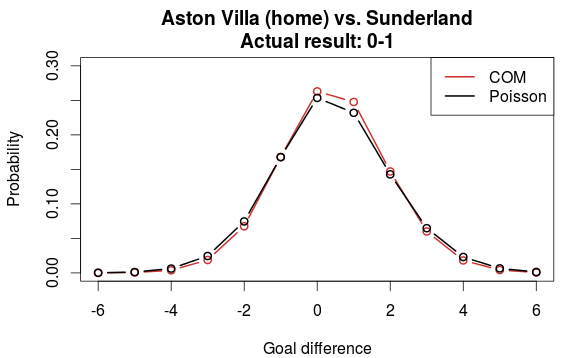Let’s compare the models using two teams from the bottom of the table, Wigan vs. Wolverhampton. Again, not much difference. Also note that there is basically no change in the probability for the actual result.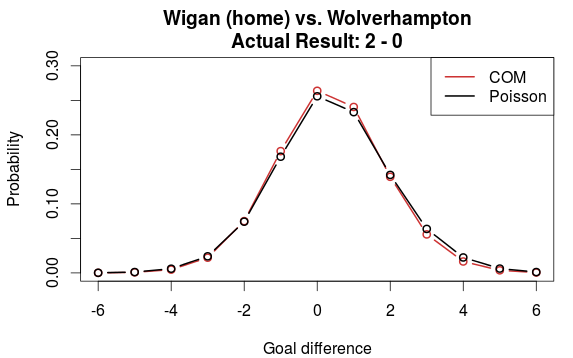OK, so far the comparisons have been based on teams of similar strengths. But take Blackburn (15th on the table) vs. Liverpool (6th). Now we see that the COM model and Poisson model differ a bit. Here the COM model does a worse prediction of the actual result compared to the Poisson model. But only considering the league positions, the skewing of the distribution in favor of Liverpool in this case may not be totally unreasonable.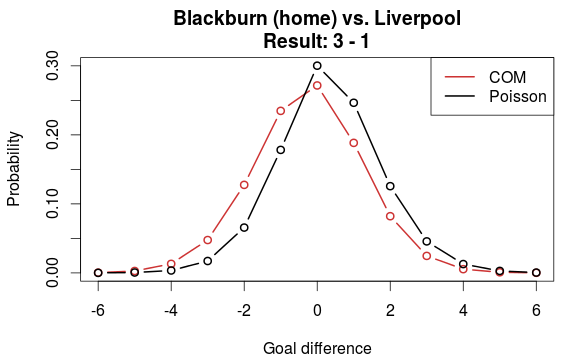The last plot is compares the distributions between Chelsea (2nd on the table) vs. Birmingham (18th). Here we clearly see that there is a substantial difference in the prediction between the two models. The COM model favors Chelsea much more than the Poisson model does, which in this case give a much higher probability for the correct result.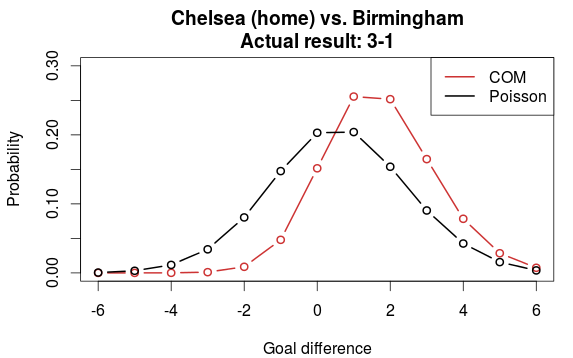From just looking at these few plots, I think we can conclude that the (underdispersed) COM model differs from the Poisson model where there is a greater difference in strength between the two sides.

Underdispersed Poisson alternatives seem to be better at predicting football results

In the previous post I discussed some Poisson-like probability distributions that offer more flexibility than the Poisson distribution. They typically have an extra parameter that controls the variance, or dispersion. The reason I looked into these distributions was of course to see if they could be useful for modeling and predicting football results. I hoped in particular that the distributions that can be underdispersed would be most useful. If the underdispersed distributions describe the data well then the model should predict the outcome of a match better than the ordinary Poisson model.

The model I use is basically the same as the independent Poisson regression model, except that the part with the Poisson distribution is replaced by one of the alternative distributions. Let the $$Y_{ij}$$ be the number of goals scored in game i by team j

$$Y_{ij} \sim f(\mu_{ij}, \sigma)$$
$$log(\mu_{ij}) = \gamma + \alpha_j + \beta_k$$

where $$\alpha_j$$ is the attack parameter for team j, and $$\beta_k$$ is the defense parameter for opposing team k, and $$\gamma$$ is the home field advantage parameter that is applied only if team j plays at home. $$f(\mu_{ij}, \sigma)$$ is one of the probability distributions discussed in the last post, parameterized by the location parameter mu and dispersion parameter sigma.

To these models I fitted data from English Premier League from the 2010-11 season to the 2014-15 season. I also used Bundesliga data from the same seasons. The models were fitted separately for each season and compared to each other with AIC. I consider this only a preliminary analysis and I have therefore not done a full scale testing of the accuracy of predictions where I refit the model before each match day and use Dixon-Coles weighting.

The five probability distributions I used in the above model was the Poisson (PO), negative binomial (NBI), double Poisson (DPO), Conway-Maxwell Poisson (COM) and the Delaporte (DEL) which I did not mention in the last post. All of these, except the Conway-Maxwell Poisson, were easy to fit using the gamlss R package. I also tried two other gamlss-supported models, the Poisson inverse Gaussian and Waring distributions, but the fitting algorithm did not work properly. To fit the Conway-Maxwell Poisson model I used the CompGLM package. For good measure I also fitted the data to the Dixon-Coles bivariate Poisson model (DC). This model is a bit different from the rest of the models, but since I have written about it before and never really tested it I thought this was a nice opportunity to do just that.

The AIC calculated from each model fitted to the data is listed in the following table. A lower AIC indicates that the model is better. I have indicated the best model for each data set in red.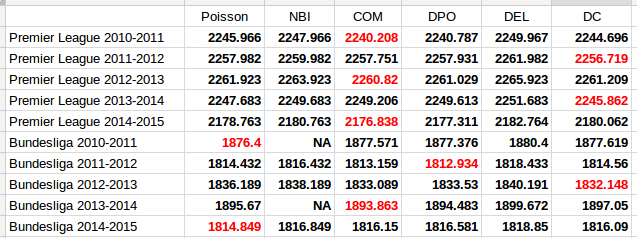The first thing to notice is that the two models that only account for overdispersion, the Negative Binomial and Delaporte, are never better than the ordinary Poisson model. The other and more interesting thing to note, is that the Conway-Maxwell and Double Poisson models are almost always better than the ordinary Poisson model. The Dixon-Coles model is also the best model for three of the data sets.

It is of course necessary to take a look at the estimates of the parameters that extends the three models from the Poisson model, the $$\sigma$$ parameter for the Conway-Maxwell and double Poisson and the $$\rho$$ for the Dixon-Coles model. Remember that for the Conway-Maxwell a $$\sigma$$ greater than 1 indicates underdispersion, while for the Double Poisson model a $$\sigma$$ less than 1 is indicates underdispersion. For the Dixon-Coles model a $$\rho$$ less than 0 indicates an excess of 0-0 and 1-1 scores and fewer 0-1 and 1-0 scores, while it is the opposite for $$\rho$$ greater than 0.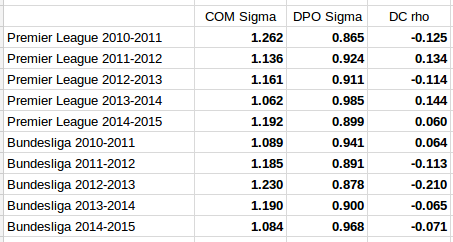It is interesting to see that the estimated dispersion parameters indicate underdispersion for all the data sets. It is also interesting to see that the data sets where the parameter estimates are most indicative of equidispersion is where the Poisson model is best according to AIC (Premier League 2013-14 and Bundesliga 2010-11 and 2014-15).

The parameter estimates for the Dixon-Coles model do not give a very consistent picture. The sign seem to change a lot from season to season for the Premier League data, and for the data sets where the Dixon-Coles model was found to be best, the signs were in the opposite direction of what where the motivation described in the original 1997 paper. Although it does not look so bad for the Bundesliga data, this makes me suspect that the Dixon-Coles model is prone to overfitting. Compared to the Conway-Maxwell and double Poisson models that can capture more general patterns in all of the data, the Dixon-Coles model extends the Poisson model to just parts of the data, the low scoring outcomes.

It would be interesting to do fuller tests of the prediction accuracy of these three models compared to the ordinary Poisson model.

Some alternatives to the Poisson distribution

One important characteristic of the Poisson distribution is that both its expectation and the variance equals parameter $$\lambda$$. A consequence of this is that when we use the Poisson distribution, for example in a Poisson regression, we have to assume that the variance equals the expected value.

The equality assumption may of course not hold in practice and there are two ways in which this assumption can be wrong. Either the variance is less than the expectation or it is greater than the expectation. This is called under- and overdispersion, respectively. When the equality assumption holds, it is called equidispersion.

There are two main consequences if the assumption does not hold: The first is that standard errors of the parameter estimates, which are based on the Poisson, are wrong. This could lead to wrong conclusions when doing inference. The other consequence happens when you use the Poisson to make predictions, for example how many goals a football team will score. The probabilities assigned to each number of goals to be scored will be inaccurate.

(Under- and overdispersion should not be confused with heteroscedasticity in ordinary linear regression. Poisson regression models are naturally heteroscedastic because of the variance-expectation equality. Dispersion refers to what relationship there is between the variance and the expected value, in other words what form the heteroscedasticity takes.)

When it comes to modeling and predicting football results using the Poisson, a good thing would be if the data were actually underdispersed. That would mean that the probabilities for the predicted number of goals scored would be higher around the expectation, and it would be possible to make more precise predictions. The increase in precision would be greatest for the best teams. Even if the data were really overdispersed, we would still get probabilities that more accurately reflect the observed number of goals, although the predictions would be less precise.

This is the reason why I have looked into alternatives to the Poisson model that are suitable to model count data and that are capable of being over- and underdispersed. Except for the negative binomial model there seems to have been little focus on more flexible Poisson-like models in the literature, although there are a handful of papers from the last 15 years with some applied examples.

I should already mention the gamlss package, which is an extremely useful package that can fit a large number of regression type models in R. I like to think of it as the glm function on steroids. It can be used to create regression models for a large number of distributions (50+) and using different forms of dependent variables (for example random effects and splines) and doing regression on distribution parameters other than the usual expectation parameters.

The models that I have considered usually have two parameters. The two parameters are often not easy to interpret, but the distributions can be re-parameterized (which is done in the gamlss package) so that the parameters describe the location (denoted $$\mu$$, often the same as the expectation) and shape (denoted $$\sigma$$, often a dispersion parameter that modifies the association between the expectation and variance). Another typical property is that they equal the Poisson for certain values of the shape parameter.

As I have already mentioned, the kind of model that is most often put forward as an alternative to the Poisson is the Negative binomial distribution (NBI). The advantages of the negative binomial are that is well studied and good software packages exists for using it. The shape parameter $$\sigma > 0$$ determines the overdispersion (relative to the Poisson) so that the closer it is to 0, the more it resembles the Poisson. This is a disadvantage as it can not be used to model underdispersion (or equidispersion, although in practice it can come arbitrarily close to it). Another similar, but less studied, model is the Poisson-inverse Gaussian (PIG). It too has a parameter $$\sigma > 0$$ that determines the overdispersion.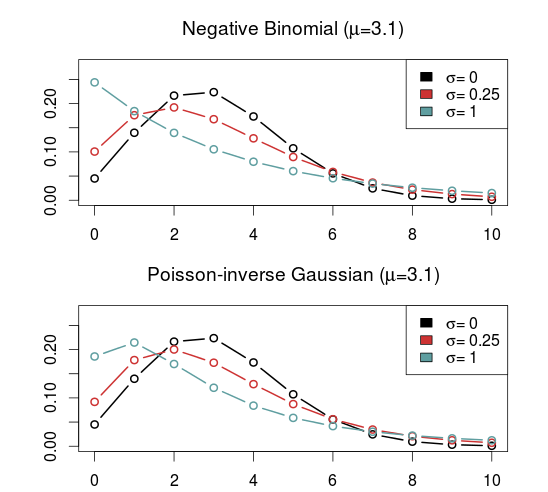A large class of distributions, called Weighted Poisson distributions, is capable of being both over- and underdispersed. (The terms Weighted in the name comes from a technique used to derive the distribution formulas, not that the data is weighted) A paper describing this class can be found here. The general form of the probability distribution is

$$P(x;\theta,\alpha)=\frac{e^{\mu x+\theta t(x)}}{x!C(\theta,\alpha)}$$

where $$t(x)$$ is one of a large number of possible functions, and $$C(\theta,\alpha)$$ is a normalizing constant which makes sure all probabilities in the distribution sum to 1. Note that I have denoted the two parameters using $$\theta$$ and $$\alpha$$ and not $$\mu$$ and $$\sigma$$ to indicate that these are not necessarily location and shape parameters. I think this and interesting class of distributions that I want to look more into, but since they are not generally implemented in any R package that I know of I will not consider them further now.

Another model that is capable of being over- and underdispersed is the Conway–Maxwell–Poisson distribution (COM), which incidentally is a special case of the class of Weighted Poisson distributions mentioned above (see this paper). The Poisson distribution is a special case of the COM when $$\sigma = 1$$, and is underdispersed when $$\sigma > 1$$ and overdispersed when $$\sigma$$ is between 0 and 1. One drawback with the COM model is that the expected value depends on both parameters $$\mu$$ and $$\sigma$$, although it is dominated by $$\mu$$. This makes the interpretation a bit difficult, but it may not be a problem when making predictions.

Unfortunately, the COM model is not supported by the gamlss package, but there are some other R packages that implements it. I have tried a few of them and the only one that I got to work is CompGLM, which for some reason does not use the location ($$\mu$$) and shape ($$\sigma$$) parameterization.The Double Poisson (DP) is another interesting distribution which also equals the Poisson distribution when $$\sigma = 1$$, but is overdispersed when $$\sigma > 1$$ and underdispersed when $$\sigma$$ is between 0 and 1. The expectation does not depend on the shape parameter $$\sigma$$, and it is approximately equal to the location parameter $$\mu$$. Another interesting thing about the Double Poisson is that it is belongs to a larger group of distributions called double exponential families which also lets you derive a binomial-like distribution with an extra dispersion parameter which can be useful in a logistic regression setting (see this paper, or this preprint).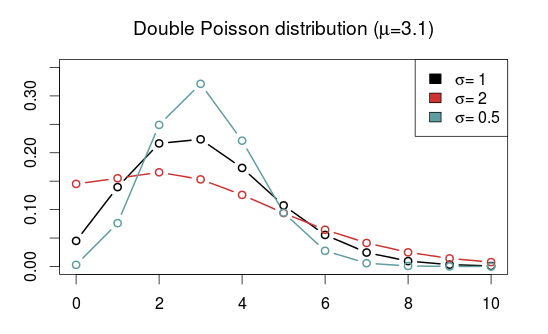In a follow up post I will try to use these distributions in regression models similar to the independent Poisson model.

A hectic schedule has some effect on the outcome of a football match

It may be that a football team who has had a hectic period with a lot of games will, because of lack of training and restitution, perform poorer. The Wikipedia page for the FA Cup mentions Manchester United’s absence from the cup as a reason for why they won the Premier League by 18 points in the 1999-2000 season. If this is indeed the case, then this is something we could try to exploit in a prediction model.

I basically used the same data and model as I have used before. I used data from the English Championship and the Premier League, and predicted the Premier League games from January 2007 until January 2015 using the independent Poisson model with the Dixon & Coles weighting method (more details on the setup here and here). In addition I constructed a new variable, the number of matches each team has played the last x number of days, were we can use and try different values of x. As a pretentious shorthand I will call this the Match Schedule Intensity Index (MSII). Matches from the FA Cup, Europa Cup and Champions League were also included in the calculations.

As usual I used the ranked probability score (RPS) to assess the prediction accuracy.

I tried four different number of days back in time: 21, 25, 28 and 31 days. I also varied the time weighing parameter $$\xi$$ a bit to see how these things varied together.

Plotting the RPS, number of days back in time and the different values of $$\xi$$ against each other gives the following: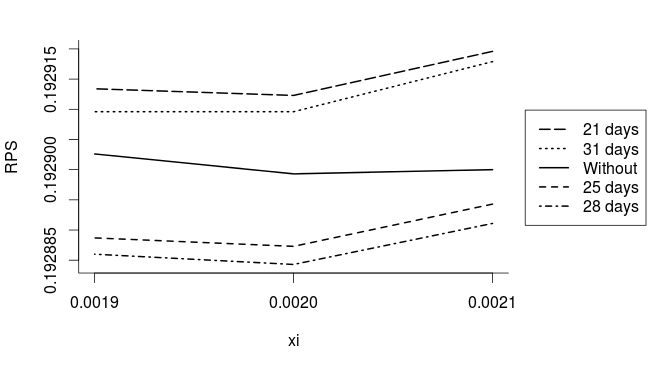We see that looking back 28 days in time gives the lowest RPS and this the most accurate predictions of the four alternatives. 25 days is almost as good as 28 days, while 21 and 31 days performs poorer than not having the MSII in the model at all. I am not sure how important the drop in RPS is, as the changes are around the 4th and 5th decimal place. It is probably not that much, but on the other hand, this is an average over 3000 matches, and the number of days back in time seems to be a more important parameter than the small changes in $$\xi$$ that I tried.

It is also interesting to see what effect the MSII has on the number of goals scored. I plotted the estimated multiplicative effect for each additional match for all the fitted models from 2007 to 2015 using the best model with 28 days and $$\xi=0.0020$$.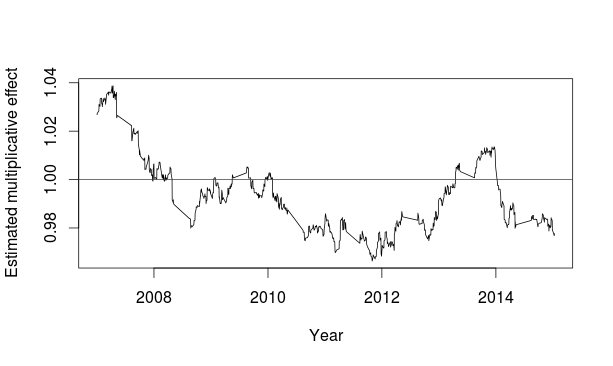I expected the effect of additional matches to be negative, meaning the more games the team has recently played, the fewer goals will they be expected to score. This seems to be at least halfway true, except for a few dips over on the positive side around 2010 and 2013-2014, and a rather large positive effect from the start in 2007 until 2008. This was a bit surprising, and I don’t know why. It would be interesting to redo the analysis with data going further back in time to see how far back the positive effect goes.

Is the effect large? Not really. The most extreme values of the multiplicative effects for the MSII is around 0.97 and 1.04. These values means that for each match a team has played more in the last four weeks they are expected to score around 3-4% more or fewer goals. This effect is around 10% for a team that has played four matches in four weeks, which is a typical mid-season schedule. This is not that big of a deal for individual matches, but it seem to improve the predictions in the long run. But I also think it is necessary to keep in mind that the effect in seems to be mostly absent in some periods.